Smartick is an advanced online program that teaches kids math and coding in only 15 min. a day

Jan12

# Mental Calculation: Horizontal Addition and Subtraction

To solve vertical additions and subtractions, we use written algorithms. We’ve all learnt how to work them out on a piece of paper. These algorithms are based on digits, and positional value does not matter. In other words, no matter whether we are adding or subtracting in the tens or thousands column, the procedure we follow is the same.

On the other hand, we cannot use this written algorithm for horizontal addition and subtraction. Trying to solve them by thinking in the same way that we would on paper is not effective. Therefore, we’re going to learn a couple of mental calculation strategies that will help when solving horizontal additions and subtractions.

The strategies we’re going to look at are based on numbers and take into account the positional value of each digit (units, tens, hundreds…).

We’ve already covered strategies for solving incomplete horizontal additions and subtractions in a previous post, involving decomposition (breaking down numbers) as a mental calculation strategy. Here we’ll learn two new strategies:

• In the first, we’ll use decomposition and continue with an addition chain.
• In the second, we will learn to subtract using the counting-up method.

### The first strategy for horizontal addition and subtraction: Decomposition + addition chain

This strategy involves breaking down the summands, paying attention to positional value, and then adding. These sums in the addition chain are more simple because they don’t mix positional values; the tens are added to the tens, the hundreds to the hundreds, etc… There’s no one way to use this strategy, each student will adapt it to their abilities and other strategies that they’ve developed.

Let’s look at an example:We can approach this addition in many different ways using the decomposition and addition chain method. We’re going to show two different possibilities that we’ll call A and B.

A1.- We break down the first summand:            2463 = 2000 + 400 +   60 + 3

And then the second summand:                             1382 = 1000 + 300 +   80 + 2

A2.- We chain add the decomposed numbers:          3000 + 700 + 140 + 5  = 3845

B1.- We break down one of the summands:        1382 = 1000 + 300 +  80 + 2

B2.- We chain add the broken down numbers to the other summand:     2463 + 1000 + 300 +  80 + 2 = 3845

### The second strategy for horizontal addition and subtraction: Counting-up

Counting-up is a mental calculation strategy for subtraction that needs prior explanation if you’ve never encountered it. In general, we understand subtraction as taking something away. The minuend is taken away from the subtrahend and we are left with the difference as the result (e.g. 10 – 6 = ?). But, it can also be understood as an ascending staircase. In this case, the difference is the amount that needs to be added to the subtrahend in order to reach the minuend. Therefore we can look at the subtraction as an addition with an unknown number (e.g. 10 – 6 = ? can be seen as 6 + ? = 10). With this in mind, it’s easy to count up from the subtrahend until you reach the minuend.

Here’s an example: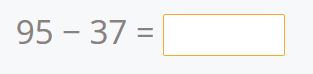It’s a mental calculation strategy, but we’ll represent it visually on an empty number line. We’ve marked the subtrahend and minuend on it: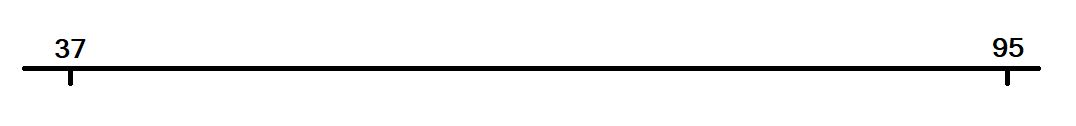Now we add a number to the subtrahend that brings us to the next ten: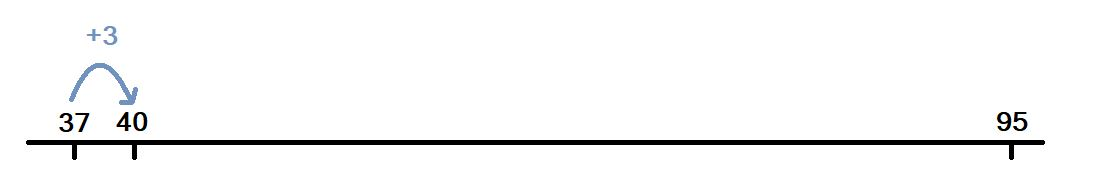Then we add another number to reach the ten before the minuend: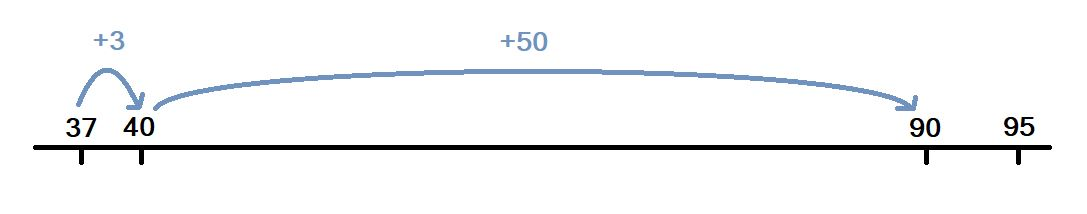And finally, we add another number to get to the minuend.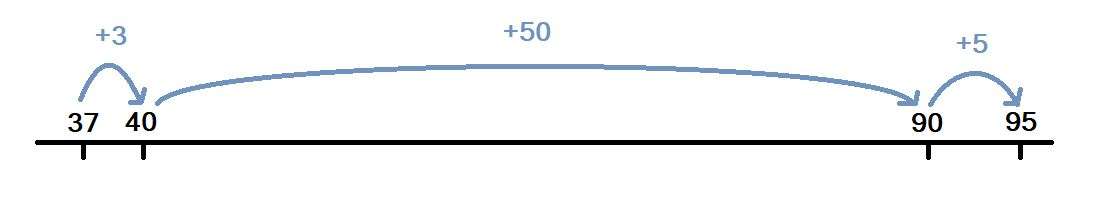Now we just have to add together the numbers we used to get from the subtrahend to the minuend:

3 + 50 + 5 = 58.

This is an open strategy that adapts to the specific abilities of each student. Each person will add the numbers in the way that best suits them. This method can also be used for subtraction with three- and four-digit numbers. As we increase the order of magnitude of the numbers, the strategy grows in complexity. It increases the possibilities of numbers to add: to reach the next ten of the subtrahend, to reach the next hundred of the subtrahend, to reach the hundred before the minuend… It needs practice to be mastered. And gradually, as students learn to count up, they simultaneously develop mental strategies for addition and subtraction.

The two mental calculation strategies for solving horizontal addition and subtraction that we’ve learned today are open and flexible. They adapt to the experience, capacity, and need of each individual. In addition, they are also combinable with other mental calculation strategies.